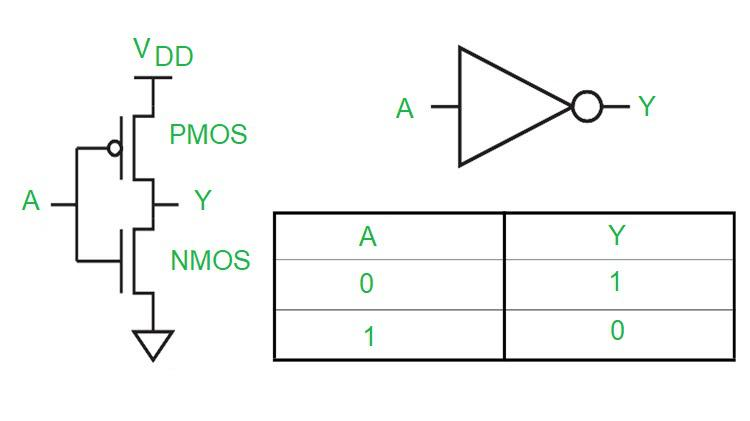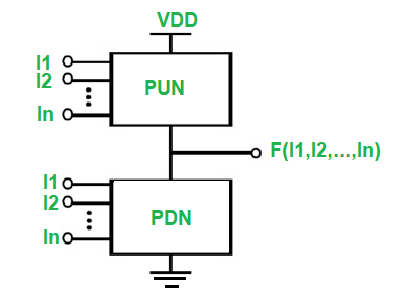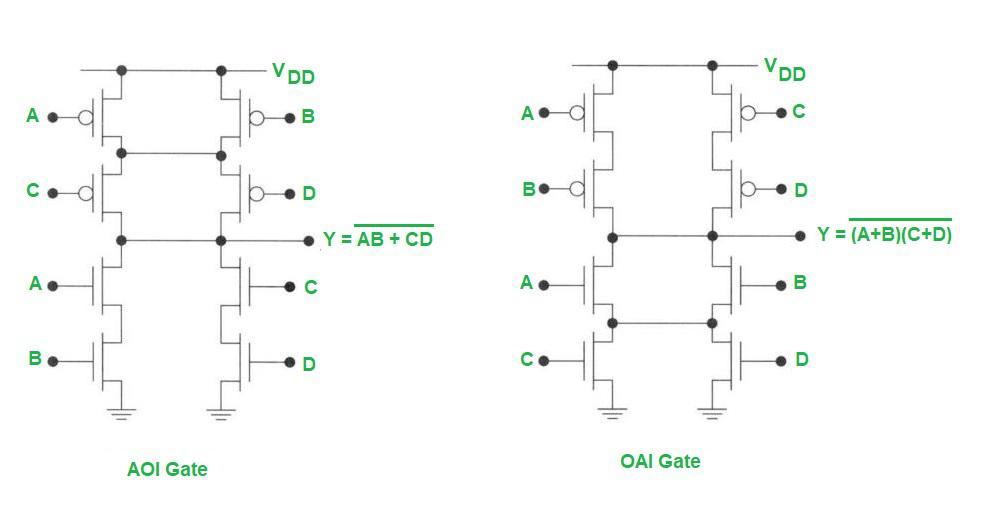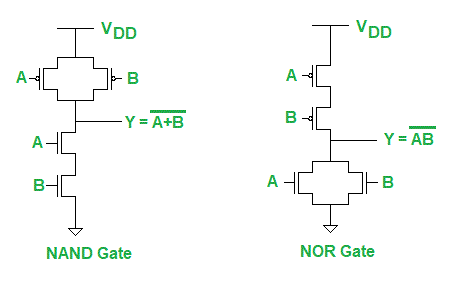Open in App
Not now

# CMOS Logic Gate

• Difficulty Level : Easy
• Last Updated : 23 Feb, 2023

The logic gates are the basic building blocks of all digital circuits and computers. These logic gates are implemented using transistors called MOSFETs. A MOSFET transistor is a voltage-controlled switch. The MOSFET acts as a switch and turns on or off depending on whether the voltage on it is either high or low. There are two types of MOSFETs: NMOS and PMOS. The NMOS turns on when the voltage is high and off when the voltage is low. The PMOS, on the other hand, turns on whenever the voltage is low and goes off as the voltage goes high. When the two are used together to realize the logic gates, they are called CMOS (Complementary MOS). The reason they are called complementary is that NMOS and PMOS work in a complementary fashion. When the NMOS switch turns on, the PMOS gets off, and vice-versa.

### CMOS Inverter:

The CMOS inverter is shown below. It consists of a series connection of a PMOS and an NMOS. VDD represents the voltage of logic 1, while the ground represents logic 0. Whenever the input is high or 1, the NMOS is switched on while the PMOS is turned off. Thus output Y is directly connected to the ground and thus comes to be logic 0. When the input is logic 0, the reverse happens – NMOS goes off and PMOS goes on. This provides a direct path between VDD and output Y. Hence Y becomes high. This is the basic principle of operation of a CMOS inverter.CMOS Inverter

From the above analysis, we can infer that for implementing any boolean function using CMOS technology, we need to make a switching circuit with PMOS switches in the upper block that turns on when its inputs are low, and NMOS switches in the lower block that turns on when its inputs are high. The two blocks must operate in a complementary sense. The upper block consisting of only PMOS is called a pull-up network (PUN) because it pulls up the output to VDD or logic high. The lower block consisting of NMOS is called a pull-down network (PDN) because it pulls down the output to ground or logic low. Any boolean function can be realized using PUN and PDN.PUN and PDN

Suppose we have Y = F(A, B, C, D). We have to obtain the PDN and PUN blocks from this given boolean expression.

For getting the PDN block, we need to obtain Y’ in terms of non-complementary variables A, B, C, and D. If we have AND in the expression of Y’, then it means two NMOS in series to ground. If there is an OR, it means two NMOS in parallel.

For the PUN, we need Y in terms of complemented variables A’, B’, C’, and D’. Again here if we have AND in the expression of Y, we need two PMOS in series, and an OR means two PMOS in parallel.

### AOI Gate and OAI Gate:

AOI (and-or-invert) and OAI (or-and-invert) gates are two basic configurations that can be realized using CMOS logic. The CMOS realization of these two types of gates is shown below. Note that the two gates are dual to each other. The PDN of the AOI gate is structurally similar to the OAI gate’s PUN, and the AOI gate’s PUN is structurally similar to the PDN of the OAI gate.AOI Gate and OAI Gate

### NAND Gate and NOR Gate:

NAND and NOR gates can be easily realized using CMOS logic as shown below.NAND Gate and NOR Gate

AND and OR gates are usually realized by using a NAND gate in series with a NOT gate, or a NOR gate in series with a NOT gate.

My Personal Notes arrow_drop_up
Related Articles